It looks like you're using Internet Explorer 11 or older. This website works best with modern browsers such as the latest versions of Chrome, Firefox, Safari, and Edge. If you continue with this browser, you may see unexpected results.

# Introduction to statistics: Inferential statistics

Introduction to statistics## What are inferential statistics?

So far we have been using descriptive statistics to describe a sample of data, by calculating sample statistics such as the sample mean ($$\bar{x}$$) and sample standard deviation ($$s$$).

However research is often conducted with the aim of using these sample statistics to estimate (and compare) true values for populations. The latter are known as population parameters, and are denoted by Greek letters such as $$\mu$$ (population mean) and $$\sigma$$ (population standard deviation). Inferential statistics allow us to make statements about unknown population parameters, based on sample statistics obtained for a random sample of the population.

There are two key types of inferential statistics, and these will both be covered on this page. Their definitions are as follows:

Before we look at estimation and hypothesis testing however, it is important to note that a random sample from a population is only one of a number of possible samples, and that values for sample statistics (i.e. the sample mean) can, in theory, be calculated for each possible sample of the same size. Hence a sample statistic has a distribution of its own which is known as the sampling distribution, and this is an important concept in inferential statistics.

Sampling distributions of sample statistics from random samples have these properties:

• as the sample size increases, the shape of the sampling distribution becomes increasingly similar to the normal distribution (this is the Central Limit Theorem);
• the mean of the sampling distribution equals the mean of the population; and
• the standard deviation of the sampling distribution is the standard error.

Before proceeding to the next section, test your understanding of inferential statistics by choosing the best answer for the following question:

## Estimation

Point estimates

Suppose a researcher is interested in cholesterol levels in a population. If they recruit a sample randomly from the population, they can estimate the cholesterol level of the whole population using the actual cholesterol level they calculated directly from the sample.

In this case:

• the sample mean cholesterol level ($$\bar{x}$$) is a point estimate of the population mean cholesterol level ($$\mu$$); and
• the sample standard deviation for cholesterol level ($$s$$) is a point estimate of the population standard deviation ($$\sigma$$).

While point estimates are useful, often it is preferable to estimate a population parameter using a range of values, so that the likely variation between the sample and population statistics is taken into account. This is where confidence intervals come in...

Confidence intervals

A confidence interval gives a range of values as an estimate for a population statistic, along with an accompanying confidence coefficient. This is the level of certainty that the interval includes the population statistic, and is typically $$95\%$$. For example, a $$95\%$$ confidence interval for population mean blood glucose level of ($$4$$ mmol/L, $$6$$ mmol/L) indicates that we are $$95\%$$ certain that the population mean blood glucose level lies between $$4$$ mmol/L and $$6$$ mmol/L.

For another way of interpreting confidence intervals, think back to the sampling distribution of a sample statistic and consider that you could calculate a confidence interval for each possible sample of the same size. A $$95\%$$ (for example) confidence interval means that you would expect 95 out of every 100 of these intervals to contain the population mean.

Before calculating a confidence interval for a population you should ensure that the following assumptions are valid:

If these assumptions are valid, a confidence interval can be calculated based on the sampling distribution of the sample statistic according to the general formula: $$\textrm{confidence interval} = \textrm{sample statistic} \pm \textrm{a multiple of the standard error of the statistic}$$

Note that the multiple to use in the formula above depends on the confidence coefficient used, with the most common confidence coefficient of $$95\%$$ requiring a multiple of $$1.96$$ (this relates back to the normal distribution, and the fact that $$95\%$$ of the area under a normal curve lies within 1.96 standard deviations of the mean). Note also that the standard error is calculated differently for different sample statistics, and for example that the standard error for the sample mean for a sample of size $$n$$ with standard deviation $$s$$ is $$\frac{s}{\sqrt{n}}$$.

Hence the formula to calculate the $$95\%$$ confidence interval for the population mean using a sample of size $$n$$ with mean $$\bar{x}$$ and standard deviation $$s$$ is: $$95\% \textrm{ confidence interval for population mean} = \bar{x} \pm 1.96 \times \frac{s}{\sqrt{n}}$$

Some important points to note about confidence intervals are as follows:

• the interval is symmetric about the sample statistic,
• the length of the interval increases for higher levels of confidence,
• the length of the interval is shorter for larger samples than for smaller samples,
• the larger the sample size, the closer our estimate is likely to be to the population value; and
• wider intervals are obtained from variables with larger standard deviations since more variation in the variable implies less accuracy in estimation.

Finally, it is important to remember that the population parameter is fixed and it is the sample mean and interval that change from sample to sample. Once the interval is calculated then the unknown population value is either inside or outside of the interval, and we can only state the certainty with which we believe the interval to contain the population value.

If you would like to practise calculating and interperting confidence intervals, have a go at one or both of the following activities.

Activity 1

Activity 2

## Hypothesis Testing

Hypothesis testing involves formulating hypotheses about the population in general based on information observed in a sample. These hypotheses can then be tested to find out whether differences and relationships observed in the sample are statistically significant and/or practically significant in terms of the population.

In order to do this, two complementary, contradictory hypotheses need to be formulated; the null hypothesis and the alternative hypothesis (or research hypothesis). Definitions of these are as follows:

Both hypotheses can be written using either words or symbols, often in a few different ways. For example if we want to test whether a new drug has significantly reduced the mean blood pressure of a population of patients, some example hypotheses are:

$$\textrm{H}_\textrm{0}$$: there is no significant difference in blood pressure before and after the drug
$$\textrm{H}_\textrm{0}: \mu_{\textrm{bp before}} = \mu_{\textrm{bp after}}$$
$$\textrm{H}_\textrm{0}: \mu_{\textrm{bp before}} - \mu_{\textrm{bp after}} = 0$$

$$\textrm{H}_\textrm{A}$$: there is a significant difference in blood pressure before and after the drug
$$\textrm{H}_\textrm{A}: \mu_{\textrm{bp before}} \neq \mu_{\textrm{bp after}}$$
$$\textrm{H}_\textrm{A}: \mu_{\textrm{bp before}} - \mu_{\textrm{bp after}} \neq 0$$

If you would like to practise writing hypotheses, have a go at formulating null and alternative hypotheses for the following examples:

Once the hypotheses have been formulated they can then be tested for statistical and practical significance, as detailed in the following sections.

Statistical significance

In order to test for statistical significance an appropriate test needs to be conducted (some common examples of which are covered in later sections of this page), which will produce a test statistic. This test statistic compares the value of the sample statistic (for example, the sample mean change in blood pressure in our blood pressure example) with the value specified by the null hypothesis for the population statistic (i.e. mean change in blood pressure of zero). Therefore a large test statistic indicates that there is a large discrepancy between the hypothesised value and the sample statistic - although note that the test statistic is not simply equal to the difference between them, and that the sample standard deviation and sample size are also involved in its calculation.

The test will also produce a $$p$$ value, which is the probability of obtaining the test statistic in question if the null hypothesis is true. It is this value that is interpreted when deciding whether or not to reject the null hypothesis, and in particular a small $$p$$ value indicates that there is a low probability of obtaining the result if the null hypothesis is true. This is evidence to reject the null hypothesis, and hence of statistical significance.

In order to decide when to reject the null hypothesis we need to choose a level of significance, denoted by $$\alpha$$. This tells us exactly how small our $$p$$ value can be before we reject the null hypothesis, and is typically $$.05$$ ($$5\%$$). Note that if:

• $$p$$ value $$\leqslant \alpha$$: There is less than or equal to $$\alpha\%$$ chance that the discrepancy between our sample statistic and our hypothesised population statistic could have occurred if the null hypothesis is true. So we REJECT the null hypothesis in favour of the alternative hypothesis, meaning that the difference or relationship we have hypothesised about is statistically significant.
•

• $$p$$ value $$> \alpha$$: There is greater than $$\alpha\%$$ chance that the discrepancy between our sample statistic and our hypothesised population statistic could have occurred if the null hypothesis is true. So we CANNOT REJECT the null hypothesis, meaning that the difference or relationship we have hypothesised about is not statistically significant.

If you would like to practise interpreting $$p$$ values, have a go at the following activity:

It is important to note here that because hypothesis testing involves drawing conclusions about complete populations from incomplete information, it is always possible that an error might occur when deciding whether or not to reject a null hypothesis. In particular there are two types of possible errors, and details of these (including how to mitigate against them) are provided below:

If you would like to test your understanding of the different error types, have a go at the following questions:

One last item of note on the concept of statistical significance, is that confidence intervals can also be used to decide whether a difference or relationship is statistically significant or not.

For example, based on data collected in the sample for our blood pressure example, a confidence interval can be calculated giving the range of values we expect the difference in mean blood pressure to lie between for the population.

If this confidence interval does not contain the value $$0$$, it means we are $$95\%$$ confident that the difference between the two values is not zero; which again means the difference is statistically significant. Confidence intervals are good because not only do they tell us about statistical significance, but they also tell us about the magnitude and direction of any difference (or relationship).

If you would like to test your understanding of this concept, have a go at these questions:

Practical significance

A slight issue with statistical significance is that it is influenced by sample size; meaning that in a very large sample, very small differences may be statistically significant, and in a very small sample, very large differences may not be statistically significant.

For this reason it is a often a good idea to measure practical significance as well, which is determined by calculating an effect size. The effect size provides information about whether the difference or relationship is meaningful in a practical sense (i.e. in real life), and it is calculated differently for different tests. Details on how to calculate effect size are covered for each of the tests outlined in subsequent sections.

## Parametric and non-parametric tests

Different inferential statistical tests are used depending on the nature of the hypothesis to be tested, and the following sections detail some of the most common ones. First, though, it is important to understand that there are two different types of tests:

As a general rule, if it’s possible to use a parametric test then these are considered preferable, as parametric tests use the mean and standard deviations in their calculations whereas non-parametric tests use the ordinal position of data. So just like the mean is typically the go-to measure of central tendency over the median, so too are parametric tests over non-parametric tests.

This following sections detail five of the most commonly used parametric tests (with reference to the non-parametric versions), and one commonly used non-parametric test.

## One sample $$t$$ test

A one sample $$t$$ test is used to test whether the sample mean of a continuous variable is significantly different to a ‘test value’ (some hypothesised value). For example, you would use it if you had a sample of student final marks and you wanted to test whether they came from a population where the mean final mark was equal to a previous year’s mean of 70. In this case the hypotheses would be:

$$\textrm{H}_\textrm{0}$$: The sample comes from a population with a mean final mark of $$70$$ ($$\mu_{\textrm{final mark}} = 70$$)
$$\textrm{H}_\textrm{A}$$: The sample does not come from a population with a mean final mark of $$70$$ ($$\mu_{\textrm{final mark}} \neq 70$$)

Before conducting a one sample $$t$$ test you need to check that the following assumptions are valid:

If the last assumption of normality is violated, or if you have an ordinal variable rather than a continuous one (such as final grades of F, $$5$$, $$6$$, $$7$$, $$8$$, $$9$$, $$10$$), the one sample Wilcoxon signed rank test should be used instead.

Assuming the assumptions for the one sample $$t$$ test are met though, and the test is conducted using statistical software, the results should look something like the following: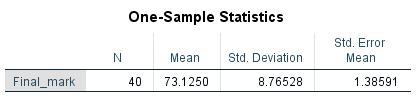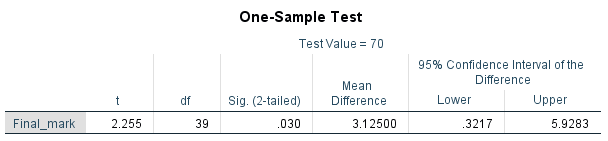Note that the first of these tables displays the descriptive statistics, which you should observe first in order to get an idea of what is happening in the sample. For example, the sample mean is $$73.125$$ as compared to the test value of $$70$$. To test whether or not this difference is statistically significant requires the second table though, and in particular the $$p$$ value and confidence interval for the difference. In terms of the $$p$$ value:

• If $$p \leqslant .05$$ we reject $$\textrm{H}_\textrm{0}$$, meaning the sample has come from a population with a mean significantly different to the test value.
• If $$p > .05$$ we do not reject $$\textrm{H}_\textrm{0}$$, meaning the sample has come from a population with a mean that is not significantly different to the test value.

In this case, our $$p$$ value of $$.03$$ means that the difference is statistically significant.

This is confirmed by the confidence interval of ($$.3217$$, $$5.9283$$) for the difference between the population mean and our test value ($$70$$); because this confidence interval does not contain zero it again means the difference is statistically significant. In fact, we are $$95\%$$ confident that the true population mean is between $$.3217$$ and $$5.9283$$ points higher than our test value.

If you would like to practise interpreting the results of a one sample $$t$$ test for statistical significance, have a go at one or both of the following examples:

Example 1:

Example 2:

Finally, to evaluate practical significance in situations where a one sample $$t$$ test is appropriate, Cohen’s $$d$$ can be used to measure the effect size. It determines how many standard deviations the sample mean is from the test value, and can be calculated as follows (recall that $$\bar{x}$$ represents the sample mean, and $$s$$ the sample standard deviation): $$\frac{\bar{x} - \textrm{test value}}{s}$$

In this case our sample mean was $$73.125$$ and our sample standard deviation was $$8.765$$, meaning our Cohen’s $$d$$ is: $$\frac{(73.125 −70)}{8.765} = 0.357$$

This is considered a small to medium effect (a Cohen's $$d$$ of magnitude $$0.2$$ is considered small, $$0.5$$ medium and $$0.8$$ or above large).

If you would like to practise measuring effect size with Cohen's $$d$$, have a go at one or both of the following examples:

Example 1:

Example 2:

## Paired samples $$t$$ test

A paired samples $$t$$ test is used to test whether there is a significant difference between sample means for continuous variables for two related groups. For example, you would use it if you had a sample of individuals who had their heart rate recorded twice; before and after exercise. In this case the hypotheses would be:

$$\textrm{H}_\textrm{0}$$: There is no significant difference in heart rate before and after exercise ($$\mu_{\textrm{HR before}} = \mu_{\textrm{HR after}}$$, or $$\mu_{\textrm{HR before}} - \mu_{\textrm{HR after}} = 0$$)
$$\textrm{H}_\textrm{A}$$: There is a significant difference in heart rate before and after exercise ($$\mu_{\textrm{HR before}} \neq \mu_{\textrm{HR after}}$$, or $$\mu_{\textrm{HR before}} - \mu_{\textrm{HR after}} \neq 0$$)

Before conducting a paired samples $$t$$ test you need to check that the following assumptions are valid:

If the last assumption of normality is violated, or if you have ordinal variables rather than continuous ones (such as blood pressures recorded as low, normal or high), the Wilcoxon signed rank test should be used instead.

Assuming the assumptions for the paired samples t test are met though, and the test is conducted using statistical software, the results should look something like the following: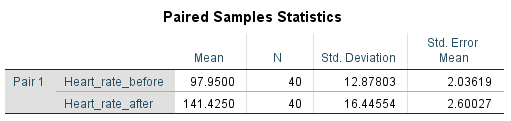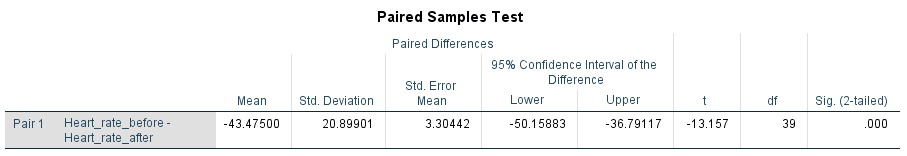Note that the first of these tables displays the descriptive statistics, which you should observe first in order to get an idea of what is happening in the sample. For example, the difference between the before and after sample means is $$43.475$$ (this value can be calculated from the means in the first table, and is also displayed in the second table- the fact that it is negative simply indicates that the heart rate after is greater than the heart rate before). To test whether or not this difference is statistically significant requires the second table though, and in particular the $$p$$ value and confidence interval for the difference. In terms of the $$p$$ value:

• If $$p \leqslant .05$$ we reject $$\textrm{H}_\textrm{0}$$, meaning the means of the two related groups are significantly different.
• If $$p > .05$$ we do not reject $$\textrm{H}_\textrm{0}$$, meaning the means of the two related groups are not significantly different.

In this case, our $$p$$ value of $$.001$$ means that the difference between the means is statistically significant.

This is confirmed by the confidence interval of ($$-50.159$$, $$-36.791$$) for the difference between the means. Because this confidence interval does not contain zero it again means the difference is statistically significant, and in fact, we are $$95\%$$ confident that the population mean heart rate after exercise is between $$36.791$$ and $$50.159$$ bpm higher than the population mean heart rate before exercise.

If you would like to practise interpreting the results of a paired samples $$t$$ test for statistical significance, have a go at one or both of the following examples:

Example 1:

Example 2:

Finally, to evaluate practical significance in situations where a paired samples $$t$$ test is appropriate, Cohen’s $$d$$ can again be used to measure the effect size. This time it measures how many standard deviations the two means are separated by, and the formula for it is as follows (where $$\bar{x}_1$$ and $$\bar{x}_2$$ represent the two sample means, and $$s_1$$ and $$s_2$$ the two sample standard deviations): $$\frac{\bar{x}_1 - \bar{x}_2}{(s_1 + s_2)/2}$$

In this case our sample means were $$97.95$$ and $$141.425$$ and our corresponding sample standards deviation were $$12.878$$ and $$16.446$$, meaning our Cohen’s $$d$$ is: $$\frac{97.95 - 141.425}{(12.878 + 16.446)/2} = −2.965$$

This is considered a very large effect (a Cohen's $$d$$ of magnitude $$0.2$$ is considered small, $$0.5$$ medium and $$0.8$$ or above large).

If you would like to practise measuring effect size with Cohen's $$d$$, have a go at one or both of the following examples:

Example 1:

Example 2:

## Independent samples $$t$$ test

An independent samples $$t$$ test is used to test whether there is a significant difference in sample means for continuous variables for two independent groups. For example, you would use it if you collected data on hours spent watching TV each week, and you wanted to test if there was a significant difference in the mean hours for males and females. In this case the hypotheses would be:

$$\textrm{H}_\textrm{0}$$: There is no significant difference in TV hours per week for males and females ($$\mu_{\textrm{males}} = \mu_{\textrm{females}}$$, or $$\mu_{\textrm{males}} - \mu_{\textrm{females}} = 0$$)
$$\textrm{H}_\textrm{A}$$: There is a significant difference in TV hours per week for males and females ($$\mu_{\textrm{males}} \neq \mu_{\textrm{females}}$$, or $$\mu_{\textrm{males}} - \mu_{\textrm{females}} \neq 0$$)

Before conducting an independent samples $$t$$ test you need to check that the following assumptions are valid:

If the last assumption of normality is violated, or if you have an ordinal variable rather than a continuous one (such as hours recorded in ranges), the Mann-Whitney U test should be used instead.

Assuming the assumptions for the independent samples t test are met though, and the test is conducted using statistical software, the results should look something like the following: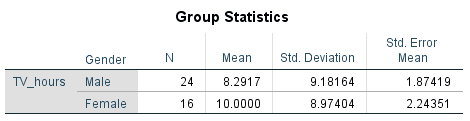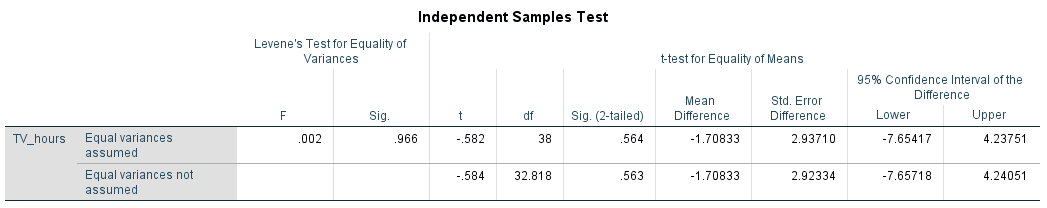Note that the first of these tables displays the descriptive statistics, which you should observe first in order to get an idea of what is happening in the sample. For example, the difference between the sample means for males and females is $$1.70833$$ (this value can be calculated from the means in the first table, and is also displayed in the second table- the fact that it is negative is simply because females watched more TV than males).

Next, note that there are actually three $$p$$ values (and two confidence intervals) in the second table; one is used in the situation where the variances for the two groups are approximately equal (the one in the middle column and top row of the table), one is used in the situation where the variances for the two groups are not approximately equal (the one in the middle column and bottom row of the table), and the other one (the first one in the table) is used to determine which situation we have.

We need to interpret the latter first; this $$p$$ value is for Levene’s Test for Equality of Variances, for which the null hypothesis is that there are equal variances. Hence, if $$p \leqslant .05$$ it is evidence to reject this null hypothesis and assume unequal variances, while if $$p > .05$$ it is evidence to fail to reject this null hypothesis and assume equal variances. Depending on which is which, determines which of the other $$p$$ values (and confidence intervals) you should interpret. In this case, the $$p$$ value of $$.966$$ means we can assume equal variances, and should interpret the $$p$$ value in the top row of the remainder of the table. Again, the interpetation of this $$p$$ value is that:

• If $$p \leqslant .05$$ we reject $$\textrm{H}_\textrm{0}$$, meaning the means of the two groups are significantly different.
• If $$p > .05$$ we do not reject $$\textrm{H}_\textrm{0}$$, meaning the means of the two groups are not significantly different.

In this case, our $$p$$ value of $$.564$$ (in the top row of the remainder of the table) means that the difference between the means is not statistically significant.

This is confirmed by the confidence interval of ($$-7.654$$, $$4.238$$) for the difference between the means. Because this confidence interval contains zero it again means the difference is not statistically significant. We are $$95\%$$ confident that the difference in mean hours spent watching TV each week for males and females is between $$-7.654$$ and $$4.238$$ hours.

If you would like to practise interpreting the results of an independent samples $$t$$ test for statistical significance, have a go at one or both of the following examples:

Example 1:

Example 2:

Finally, to evaluate practical significance in situations where an independent samples $$t$$ test is appropriate, Cohen’s $$d$$ can again be used to measure the effect size. This time it measures how many standard deviations the two means are separated by, and the formula for it is as follows (where $$\bar{x}_1$$ and $$\bar{x}_2$$ represent the two sample means, and $$s_1$$ and $$s_2$$ the two sample standard deviations): $$\frac{\bar{x}_1 - \bar{x}_2}{\sqrt{\frac{(s_1^2 + s_2^2)}{2}}}$$

In this case our sample means were $$10$$ and $$8.292$$ and our corresponding sample standards deviation were $$8.974$$ and $$9.182$$, meaning our Cohen’s $$d$$ is: $$\frac{10 - 8.292}{\sqrt{\frac{(8.974^2 + 9.182^2)}{2}}} = 0.188$$

This is considered a small effect (a Cohen's $$d$$ of magnitude $$0.2$$ is considered small, $$0.5$$ medium and $$0.8$$ or above large).

If you would like to practise measuring effect size with Cohen's $$d$$, have a go at one or both of the following examples:

Example 1:

Example 2:

## One-way ANOVA

One-way ANOVA is similar to the independent samples t test, but is used when three or more populations are compared. For example, comparing the mean weights of people who do no exercise, who do moderate exercise and who do lots of exercise.

The null hypothesis for a one-way ANOVA states that all the population means are equal, while the alternative hypothesis states that at least one of them is different.

Before conducting a one-way ANOVA you need to check that the following assumptions are valid:

If a one-way ANOVA is conducted and it turns out that at least one of the means is different, you will need to investigate further to determine where the difference lies using post hoc tests, for example Tukey's HSD.

For now though, to practise determining when the one-way ANOVA is suitable to use have a go at the following question:

Note that one-way ANOVA is just one in a family of ANOVA tests, and that other kinds of ANOVA include the following:

• Factorial ANOVA to test for differences in means between groups when there are two or more independent variables.

• One-way repeated measures ANOVA to test for differences in means between three or more related samples.

• Mixed-model ANOVA to test for differences between means when there are two or more independent variables, and you have a mixture of between subjects and within subjects variables, or of between subjects and repeated measures.

• ANCOVA (one-way analysis of covariance) to test for differences between two or more independent samples after controlling for the effects of a third variable (covariate).

• MANOVA (multivariate analysis of variance) to simultaneously test for differences between groups on multiple dependent variables.

## Chi-square test

The Chi-square test is a non-parametric test used to determine whether there is a statistically significant association between two categorical variables. For example, it could be used to test whether there is a statistically significant association between variables for gender and favourite sport. In this case the hypotheses would be:

$$\textrm{H}_\textrm{0}$$: There is no association between gender and favourite sport in the population
$$\textrm{H}_\textrm{A}$$: There is an association between gender and favourite sport in the population

Before conducting a Chi-square test you need to check that the following assumptions are valid:

Note that these first two assumptions simply require appropriate categories for both of the variables, while information for the third assumption should be provided with the results of the test (i.e. the $$0.0\%$$ below the second table below).

Assuming the assumptions for the Chi-square test are met, and the test is conducted using statistical software, the results should look something like the following: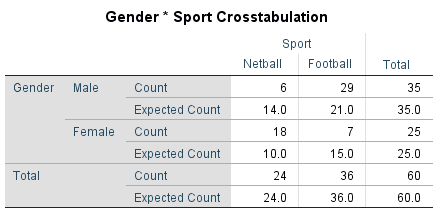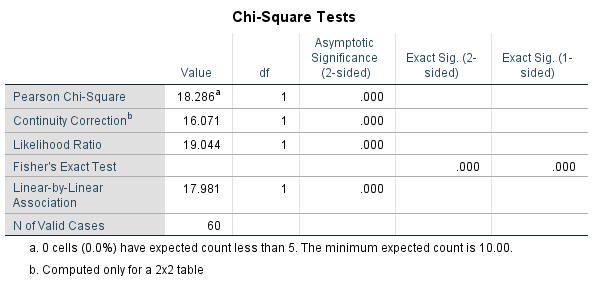Note that the first of these tables displays the descriptive statistics, which you should observe first in order to get an idea of what is happening in the sample. For example, the fact that there is a reasonably large difference between the Counts and Expected Counts indicates that there is at least some association between the variables in the sample. To test whether or not this association is statistically significant requires the second table though, and in particular the $$p$$ value for Pearson's Chi-square:

• If $$p \leqslant .05$$ we reject $$\textrm{H}_\textrm{0}$$, meaning there is a statistically significant association between the variables.
• If $$p > .05$$ we do not reject $$\textrm{H}_\textrm{0}$$, meaning there is not a statistically significant association between the variables.

In this case, our $$p$$ value of $$< .001$$ means that the association between gender and favourite sport is statistically significant.

If you would like to practise interpreting the results of a Chi-square test for statistical significance, have a go at the following example:

Finally, a few different statistics can be used for calculating effect size when a Chi squared test has been conducted, depending on the number of categories for each variable, the type of variables (nominal or ordinal) and the nature of the study. In the case where there are only two categories for each variable, then effect size can be measured using phi ($$\phi$$). This is calculated using the chi-square value ($$\chi^2$$) and the sample size ($$n$$), as follows: $$\phi = \sqrt\frac{\chi^2}{n}$$

In this case our $$\chi^2$$ value was $$18.286$$ and sample size $$60$$, meaning our $$\phi$$ is: $$\sqrt\frac{18.286}{60} = 0.552$$

This is considered a large effect (0.1 is considered a small effect size, 0.3 medium and 0.5 and above large).

Note also that an extension of $$\phi$$ for nominal variables with more than two categories is Cramer’s V, while other measures of effect size include relative risk and odds ratio.

If you would like to practise measuring effect size with $$\phi$$, have a go at the following example:

## Pearson's correlation

A hypothesis test of Pearson's correlation coefficient is used to determine whether there is a statistically significant linear correlation between two continuous variables. For example, it could be used to test whether there is a statistically significant linear correlation between heart rates before and after exercise. In this case the hypotheses would be:

$$\textrm{H}_\textrm{0}$$: There is no linear correlation between heart rates before and after exercise in the population
$$\textrm{H}_\textrm{A}$$: There is linear correlation between heart rates before and after exercise in the population

Before conducing a hypothesis test of Pearson's correlation coefficient you need to check that the following assumptions are valid:

If the variables are not normally distributed, or if the data is ordinal, you should use Spearman’s rho or Kendall’s tau-$$b$$ instead.

Assuming the assumptions for Pearson's correlation are met though, and the test is conducted using statistical software, the results should look something like the following: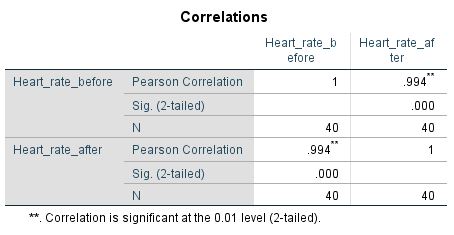This table includes the Pearson's correlation ($$r$$) value, which you should observe first in order to get an idea of what is happening in the sample. For example, the fact that it is very close to 1 indicates that there is a strong positive linear correlation between the variables in the sample. To test whether or not this linear correlation is statistically significant requires the $$p$$ value though:

• If $$p \leqslant .05$$ we reject $$\textrm{H}_\textrm{0}$$, meaning there is statistically significant linear correlation between the variables.
• If $$p > .05$$ we do not reject $$\textrm{H}_\textrm{0}$$, meaning there is not statistically significant linear correlation between the variables.

In this case, our $$p$$ value of $$< .001$$ means that the linear correlation between the before and after heart rates is statistically significant.

If you would like to practise interpreting the results of a Pearson's correlation hypothesis test for statistical significance, have a go at the following example:

Finally, note that the correlation coefficient is a measure of effect size, so a separate measure does not need to be calculated (again a value of $$0.1$$ is considered a small effect size, $$0.3$$ medium and $$0.5$$ and above large).

Furthermore, the percentage of variation in the dependent variable that can be accounted for by variation in the independent variable can be found by calculating $$r^2$$. This is known as the coefficient of determination.

In the previous example the effect size is large ($$.994$$), and the coefficient of determination of $$.988$$ indicates that $$98.8\%$$ of the variation in the after heart rate can be accounted for by variation in the before heart rate.

If you would like to practise measuring effect size for Pearson's correlation, have a go at the following example:

## Further Resources

Congratulations on making it to the end of the module! We hope you found it a useful introduction to the world of statistics. If you are interested in finding out how to conduct the statistical tests detailed in this module in the statistical software SPSS, you also might like to check out the Introduction to SPSS module.

Additionally, if you are interested in learning more about statistics, and in particular finding out about other statistical tests, you may like to make use of the following textbook:

Allen, P., Bennett, K., & Heritage, B. (2014). SPSS Statistics version 22: A practical guide. (3 ed.) Sydney: Cengage Learning Australia Pty Limited.